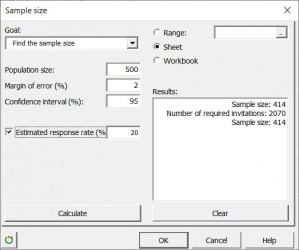# Sample Size calculator

Use this tool to calculate the number of respondents needed to obtain statistically strong results for a population or to obtain the margin of error for your sample.## Why do we need to determine the sample size for a survey?

When conducting a statistical study or a market study involving research on groups of populations, it becomes important to wonder about of the sample size. In order to obtain reliable results, it is necessary to have a sample that is as representative as possible of the population. XLSTAT makes it possible, with this tool, to calculate the right number of people to be interviewed in order to obtain a sample size that is neither too large (which would make the study more complex and expensive), or too small (which would generate erroneous results). If you already know the size of your sample, XLSTAT also allows you to check its margin of error.

## How to compute the size of a sample in XLSTAT?

Different arguments are available in the XLSTAT sample size calculator:

Goal: Choose between the calculation of the sample size and the calculation of the margin of error (depending on this choice, the following fields will be different).

Population Size: Enter the size of the study population.

Margin of error (in case of sample size calculation): Enter the margin of error that you accept for your study (in %).

Number of respondents (in the case of calculating the margin of error): Enter the number of people who responded to your survey.

Confidence interval: Enter the size of the desired confidence interval (in %).

Estimated response rate: Select this option if you want to calculate the number of invitations required to reach the correct sample size. Then enter the estimated response rate (in %).

Calculate: Click this button to display the result in the Results area of the dialog box.

## What are the results of the XLSTAT sample size calculator?

The results displayed by XLSTAT are a summary table with the population size, the margin of error, the confidence interval, the sample size, and the number of invitations required.

Waffle Chart: It allows you to represent the percentage of the sample size compared to the size of the population.

Pie Chart: It plots the sample size against the number of invitations required.### analyze your data with xlstat

14-day free trial

Included in

Related features